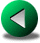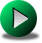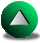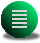Previous: Testing the Different Numerical
Next: The Wind-driven Circulation in
Up: Testing the Different Numerical

# Gravity Waves in a Square Domain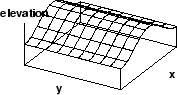In this section, we present results for the linearized SW gravity wave propagation problem in a square domain. An elevation perturbation is imposed at the beginning of the simulation, in the form of a sine wave with phase lines parallel to the y-axis: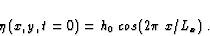(3.1)

The initial velocity is zero. The wave propagates along the x-axis. Since there is no dispersion in the y-direction, the problem simplifies to a one-dimensional problem and a simple analytical solution can be found. An equation for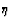only can be found by substituting the u-equation in the-equation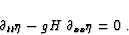(3.2)

With no normal flow boundary conditions, the solution is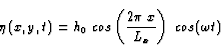(3.3)

where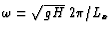. Therefore, the wave is a free mode of oscillation for the square basin. It bounces back and forth between the walls at the period of. The velocities are given by(3.4)

where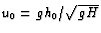.

For all models, the numerical simulation is performed up to a tenth of the characteristic period of the wave. This duration is long enough that the estimation of the effective truncation order for the different models is possible and yet not too long so that the contamination by other factors such as time discretization errors is limited. The Courant number is kept constant and is the same for all models. By increasing the resolution of the models and comparing the numerical solution to the analytical solution, we can compute the errors and the effective truncation order of each scheme. For the FD models, the grid is oriented along the walls of the square which coincide with the direction of the wave propagation, also the x-axis. Hence, there are no dispersion errors in the y-direction. However, the FE and SE methods use irregular meshes made of triangles that are randomly oriented. Therefore, these methods show a dispersion error along the y-axis which can be quantified as a function of resolution. The errors are computed and normalized as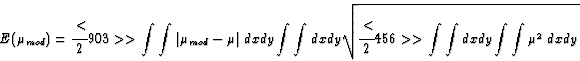(3.5)

where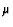and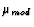represent respectively the analytical and model solution of any variable. The term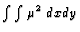is computed analytically and is therefore the same for all models. For FD models,is approximated by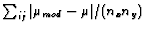. For the FE and SE models, this integral is computed by interpolating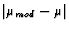onto a regular grid, summing the values and dividing by the number of sampling points. We increase the number of sampling points until a convergence criterion is satisfied. The normalized error for u is obtained from (3.5) by direct replacement ofby u. For v, this is not possible as its analytical value is zero. We have thus used the analytical value of u for. The choice of norms in (3.5) in determining the normalized error is somewhat arbitrary and other norms can be used. However the results would not be substantially different.

We first compare the accuracy of the linearized version of the 4th order A-grid model to that of the second order C-grid formulation (Figure 3.2). On this log-log plot, the slope of the curve is directly related to the order of the convergence. The C-grid scheme is very close to second order and the original A-grid model (as proposed by D93 and referred as O-FDM4) has a convergence order of close to 4. However, the errors can be reduced by a factor of six if the 4th order accuracy is extended up to the boundary (R-FDM4 version). The gradient and interpolation operators then need to be off-centered for points located less than two points away from the walls. In terms of cost, the A-grid model is very advantageous (see Fig 3.3 where only results from R-FDM4 is plotted). The extra points in the computation of the gradient operators needed for 4th order accuracy slow the model only slightly. Therefore the 4th order A-grid is cost-effective compared to the C-grid for this problem.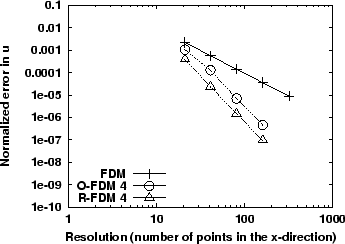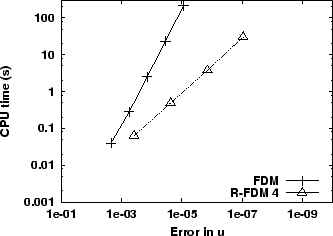For FE models, the use of irregular grids cause errors to appear in the v-component, perpendicular to the propagation direction. These errors can also be viewed as a dispersion error. One way to minimize this error would be to design meshes for which the nodes or vertices are aligned with the propagation axis (i.e. characteristic methods). Such a mesh would therefore be application dependent. We focus instead on the use of irregular meshes in which the triangles are randomly oriented since, in general ocean modelling, there are no preferential directions of propagation. We examine the four FE models introduced in Section 2.3: the Lynch and Werner lynch87,lynch91 model, the Hua and Thomasset hua84 model, the Peraire et al. peraire86 model and the Le Roux et al. leroux00 model. The respective abbreviations are: LW, HT, PZM and LLS. In our comparison study, we multiply by two the actual resolution of the mesh for the LLS model to take into account the fact that this model implicitly doubles the resolution by dividing each triangle into four sub-triangles. Figure 3.4 shows the convergence with resolution of the errors for the v- and u-components for a linearized version of all FE models. In such a case, the LLS model is plainly Eulerian instead of semi-Lagrangian. The errors are generally larger for the FE models compared to the C-grid FD model, except for the LLS model where the errors are comparable. This is notably due to the use of unstructured grids in FE models.

Table 3.1 gives the value of the convergence order for both components of the velocities for all models. The order is usually lower for the v-component (closer to first order) than that of the u-component (closer to second order) for all FE models. This is however an artefact due to studying the two components of the velocity separately. The error in v is usually smaller than the error in u. This allows for some noise contamination to lower the convergence order for vcompared to that for u. The convergence order for the velocity vector tends to be in between but closer to the convergence order for u since the errors are largest for this component.

The equal-order FE models (LW and PZM) present the best convergence order for u (about 2) and also the poorest order for v (about 1). The LLS model presents the largest order for v. The order for the HT model is closer to first than second order for both components of velocity. Theoretically, the best achievable convergence order for the FE models under consideration is second order. The fact that the convergence order for most models is less than but close to 2 for u is due to the use of irregular meshes. The change, though, is not as dramatic as predicted in Section 2.3.2 where we predict first order convergence in presence of irregular meshes for second order accurate FE formulations.

Since the LLS model is the best FE model in terms of the magnitude of the errors --to the point that the magnitude compares favorably to that of the C-grid errors-- it is worth considering some of the reasons behind this result. First, the method uses macro elements sub-divided into four elements and this may regularize'' the mesh since the four sub-triangles are identical in shape and area. Second, it is also possible that the fact that the coupled shallow water equations are reduced to coupled Helmholtz equations for the velocity improves the solution for the velocity. The fact that the order for this model is somewhat smaller compared to that of the LW and the PZM models for the u-component might be a sign that the truncation order for the pressure slightly affects the truncation order for the velocity. This will be more evident in the next test-case. For the HT model, the smaller convergence order is probably related to the use of discontinuous basis functions for the velocities, in contrast to continuous basis functions used in the other FE models. In conclusion, for this linear problem, all FE models perform relatively well --except for the HT model.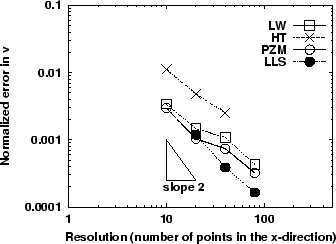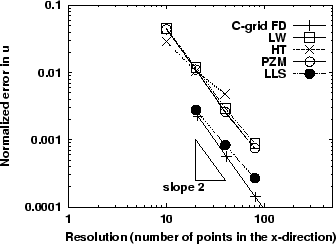We now compare the results of one FD model (C-grid) and one FE model (LW) to the discontinuous SE model (Fig 3.5 and 3.6). To make results comparable, the SE resolution (the inverse of the mean length of triangle sides) is multiplied by the maximum polynomial order. The LW-FE errors are generally larger than those of the FD and SE models. The SE model has a convergence order that varies between nc and nc-1 depending on the velocity components. If the basis functions were continuous, the best achievable convergence order would be nc+1. The loss of more than one order is probably related to the use of unstructured meshes and the fact that the basis functions are discontinuous between elements. At nc=3, the accuracy of the SE model is slightly better than the FD model. At the same resolution, the higher-order method is always more accurate (nc=5 and 7). Finally we noted that as for the FE models, the SE model shows a difference (Table 3.1) in the convergence order for v and u, with the order for v being smaller than that for u. This is related to the use of irregular grids and noise contamination problems.

Figure 3.7 shows the variation of the CPU cost with respect to the accuracy for the C-grid FD model and one A-grid FD (R-FDM4) model, the LW and LLS FE models and the discontinuous SE model. The curve is usually a straight line. The less the slope of the curve, the more accurate for the same cost one model is. The model whose curves lies on the right (left) of the others is the most (less) economic model. There is of course the possibility that some models perform better than the others depending on the range of the required accuracy due to the existence of cross-over points between the different curves. The LW model is always less accurate for the same cost with the slope being equivalent to that of the finite difference model. The LLS model enhanced accuracy compared to the other FE models (Fig. 3.4) is traded off by a large increase of the CPU cost, to the point that the LLS model is only marginally better than the LW model. The SE model with nc=5 behaves similarly to the 4th order A-grid model. However, the A-grid model is slightly more accurate for the same cost. Nonetheless, the SE model with nc=7 give better results than this 4th order FD model. From this linear test case, we conclude that it is more effective to use higher order methods (the SE and R-FDM4 models).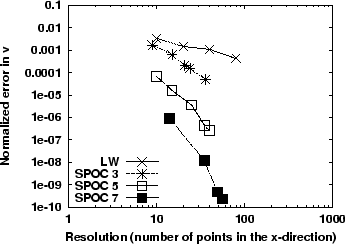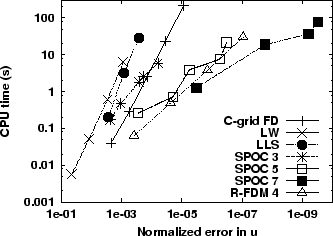Model Convergence order for the error in v Convergence order for the error in u C-grid FD - 2.03 O-FDM 4 - 3.85 R-FDM 4 - 4.09 LW 0.94 1.91 HT 1.08 1.30 LLS 1.43 1.69 PZM 1.01 1.97 SPOC 3 2.57 2.73 SPOC 5 4.00 4.68 SPOC 7 5.96 6.72Previous: Testing the Different Numerical
Next: The Wind-driven Circulation in
Up: Testing the Different Numerical

Frederic Dupont
2001-09-11